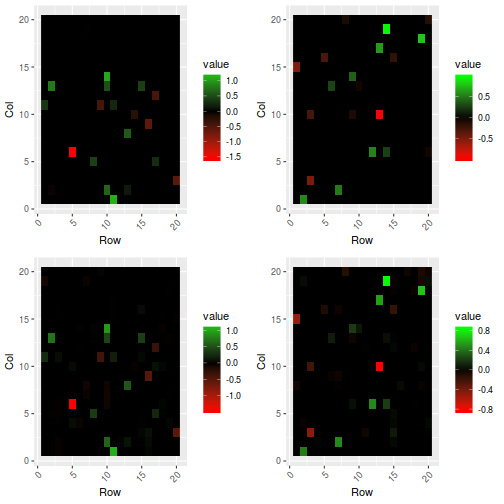# Introduction

sparsevar is an R package that estimates sparse VAR and VECM model using penalized least squares methods (PLS): it is possible to use various penalties such as ENET, SCAD or MC+ penalties. The sparsity parameter can be estimated using cross-validation or time slicing. When using ENET it is possible to estimate VAR(1) of dimension up to 200, while when using one of the other two is better not to go beyond 50. When estimating a VAR($$p$$) model then the limits are roughly $$200/p$$ and $$50/p$$, respectively.

The authors of sparsevar are Monica Billio, Lorenzo Frattarolo and Simone Vazzoler and the R package is mantained by Simone Vazzoler. This vignette describes the usage of sparsevar in R.

# Installation

The simplest way to install the package is by using the CRAN repositories, by typing in the R console

install.packages("sparsevar", repos = "http://cran.us.r-project.org")


It is also possible to install the developing version of the package by typing

install.packages("devtools", repos = "http://cran.us.r-project.org")
devtools::install_github("svazzole/sparsevar")


# Quick start

To load the sparsevar package simply type

library(sparsevar)


Using a function included in the package, we simply generate a $$20\times 20$$ VAR$$(2)$$ process

set.seed(1)
sim <- simulateVAR(N = 20, p = 2)


and we can estimate the matrices of the process using

fit <- fitVAR(sim\$series, p = 2)


The results can be seen by plotting the matrices

plotVAR(sim, fit)# Description of the package's functions

## Estimation of a VAR or VECM models

Use fitVAR for VAR model estimation or fitVECM for VECM estimation.

The common arguments for the two functions are:

• data: a matrix containing the multivariate time series (variables in columns, observations in rows);
• p: the order of the VAR model to be estimated; default p = 1 for fitVAR and p=2 for fitVECM.
• method: the method used to estimate the sparsity parameter. Default is method = "cv" (cross-validation). Another possibility is method = "timeSlice".
• penalty: the penalty used in least squares. Possible values are: "ENET", "SCAD" or "MCP";
• ...: sequence of options. Some of them depend on the penalty used, some on the method and some are global.

### Global options

• parallel: TRUE or FALSE (default). Parallel cross-validation (on the folds);
• ncores: if parallel = TRUE then you must specify the number of cores used for the parallelization (default = 1).
• nfolds: number of folds to use in the cross validation (default nfolds = 10)
• threshold: TRUE or FALSE (default). If TRUE all the elements of the VAR/VECM matrices that are small “enough” are set to 0.

### Options for penalty = "ENET"

• lambda: "lambda.min" (default) or "lambda.1se";
• alpha: a value in (0,1) (default alpha = 1). alpha = 1 is LASSO regression, alpha = 0 is Ridge LS;
• type.measure: "mse" (default) or "mae";
• nlambda: number of lambdas used for cross validation.
• foldsID: the vector containing the IDs for the folds in the cross validation.

### Options for penalty = "SCAD" or "MCP"

• eps: convergence tolerance
• picasso: TRUE or FALSE. If TRUE uses the picasso package for SCAD estimation.

### Output

The output of the function fitVAR is a S3 object of class var containing:

• mu: a vector for the mean;
• A: a list of length p containing the matrices estimated for the VAR(p) model;
• lambda: the estimated sparsity parameter;
• mse: the mean square error of the cross validation or time slicing;
• time: elapsed time for the estimation;
• series: the transformed data matrix (centered or scaled);
• residuals: the matrix of the estimated residuals;
• sigma: the variance/covariance matrix of the residuals;
• penalty: the penalty used (ENET, SCAD or MCP);
• method: the method used ("cv" or "timeSlice").

## Simulation of VAR models

Use simulateVAR. The parameters for the function are:

• N: the dimension of the process;
• nobs: the number of observations of the process;
• rho: the variance/covariance “intensity”;
• sparsity: the percentage of non zero elements in the matrix of the VAR;
• method: "normal" or "bimodal".

## Estimation of Impulse Response function

Use the functions impulseResponse and errorBands to compute the impulse response function and to estimate the error bands of the model respectively.

irf <- impulseResponse(fit)
eb <- errorBandsIRF(fit, irf)


# Examples

## Estimations' examples

results <- fitVAR(rets)


will estimate VAR(1) process using LASSO regression on the dataset rets.

The command

results <- fitVAR(rets, p = 3, penalty = "ENET", parallel = TRUE,
ncores = 5, alpha = 0.95, type.measure = "mae",
lambda = "lambda.1se")


will estimate a VAR(3) model on the dataset rets using the penalty "ENET" with alpha = 0.95 (between LASSO and Ridge). For the cross validation it will use "mae" (mean absolute error) insteadof mean square error and it will choose as model the one correspondent to the lambda which is at 1 std deviation from the minimum. Moreover it will parallelize the cross validation over 5 cores.

## IRF example

Here we compute the IRF for the model estimated in the Quick Start section.

irf <- impulseResponse(fit)
eb <- errorBandsIRF(fit, irf, verbose = FALSE)
plotIRFGrid(irf, eb, indexes = c(11,20))


## Simulations' examples

sim <- simulateVAR(N = 100, nobs = 250, rho = 0.75, sparsity = 0.05, method = "normal")